## Info

Publication number
JP4602179B2
JP4602179B2 JP2005197904A JP2005197904A JP4602179B2 JP 4602179 B2 JP4602179 B2 JP 4602179B2 JP 2005197904 A JP2005197904 A JP 2005197904A JP 2005197904 A JP2005197904 A JP 2005197904A JP 4602179 B2 JP4602179 B2 JP 4602179B2
Authority
JP
Japan
Prior art keywords
signal
means
phase
frequency
frequency spectrum
Prior art date
Legal status (The legal status is an assumption and is not a legal conclusion. Google has not performed a legal analysis and makes no representation as to the accuracy of the status listed.)
Active
Application number
JP2005197904A
Other languages
Japanese (ja)
Other versions
JP2006258786A (en
Inventor

Original Assignee

Priority date (The priority date is an assumption and is not a legal conclusion. Google has not performed a legal analysis and makes no representation as to the accuracy of the date listed.)
Filing date
Publication date
Priority to JP2005037838 priority Critical
Application filed by 三菱電機株式会社 filed Critical 三菱電機株式会社
Priority to JP2005197904A priority patent/JP4602179B2/en
Publication of JP2006258786A publication Critical patent/JP2006258786A/en
Application granted granted Critical
Publication of JP4602179B2 publication Critical patent/JP4602179B2/en
Application status is Active legal-status Critical
Anticipated expiration legal-status Critical

## Images

•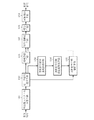•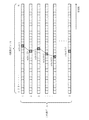•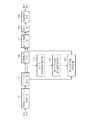•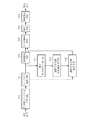•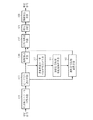•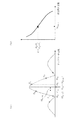•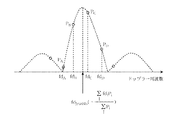•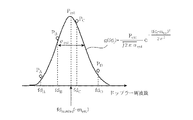•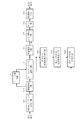•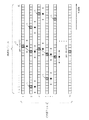•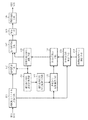•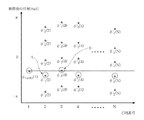•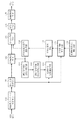•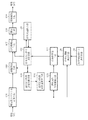•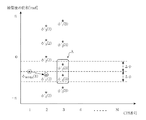•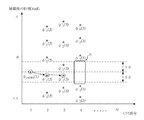•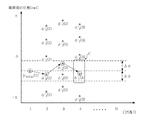•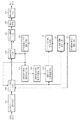•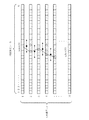••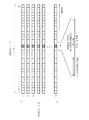•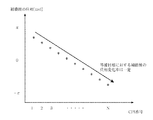•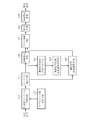•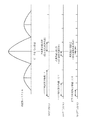•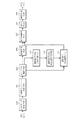•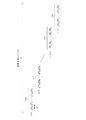•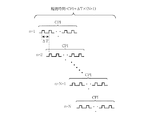## Description

The present invention relates to a radar apparatus that compensates for a phase change accompanying movement of a target and integrates a signal after phase compensation again coherently.

Conventional radar devices include the following. That is, the received signal is subjected to Fast Fourier Transform (FFT) processing for each number of specific pulse hits, and a relationship (phase curve) between pulse hit and phase change is obtained for each range bin for each output signal after FFT processing. . Next, from the phase curves obtained for each range bin, the output signal closest to the presumed motion phase curve is selected as the target signal candidate, and the phase compensation amount calculated based on the presumed motion Thus, after the phase change of the candidate signal is compensated, the signal after phase compensation is added to improve the detection performance (see, for example, Patent Document 1).

According to such a method, since amplitude information and phase information can be used, a signal to noise ratio (SNR) is improved as compared with an integration method using only amplitude information represented by non-coherent integration. As a result, an improvement in detection performance can be expected.

Japanese Patent No. 2737434

However, in the conventional radar apparatus, since the output signal closest to the assumed motion phase gradient is selected as the target candidate, it is necessary to assume the target motion in advance, and it is difficult to improve the detection performance for an unexpected motion target. There was a problem.

The present invention has been made to solve the above-described problems, and an object of the present invention is to obtain a radar apparatus capable of improving the detection performance for a high maneuver target for which it is difficult to assume a motion in advance.

The radar apparatus according to the present invention measures the relative speed corresponding to the generation frequency of the peak signal at which the intensity of the frequency spectrum of the received signal is maximum by the relative speed measuring means, and is a time during which coherent processing can be performed based on the relative speed. The phase change amount for each interval is calculated by the phase change amount calculation means. Furthermore, the phase of the peak signal is compensated by the selection signal phase compensation means using the phase change amount, and the phase signal compensated from the compensated phase and the amplitude value of the peak signal is reconstructed, and the integration means is used. A plurality of phase-compensated peak signals generated at every time interval capable of coherent processing are added.

The radar apparatus according to the present invention calculates a phase change amount for each time interval based on a relative speed corresponding to a frequency at which the peak signal is generated, and performs phase compensation using the phase change amount to reconstruct the peak signal. As a result, the radar apparatus can improve the detection performance for a high maneuvering target for which it is difficult to assume a motion in advance.

Embodiment 1 FIG.
1 is a block diagram showing a radar apparatus according to Embodiment 1 of the present invention.
As shown in the figure, the radar apparatus according to the first embodiment includes a frequency spectrum calculation unit 101, a peak signal selection unit 102, a selection signal relative speed measurement unit 103, a selection signal phase change amount calculation unit 104, a selection signal phase compensation unit 105, An amplitude calculation unit 106, a signal reconstruction unit 107, an integration unit 108, and a threshold detection unit 109 are provided.

The frequency spectrum calculation unit 101 has a function of obtaining the frequency spectrum of the received signal input at every time interval that allows coherent processing. Hereinafter, a time interval in which coherent processing can be performed is referred to as CPI (Coherent Processing Interval). The peak signal selection unit 102 has a function of selecting a peak signal having the maximum frequency spectrum intensity with respect to the frequency spectrum obtained by the frequency spectrum calculation unit 101. The selection signal relative speed measuring means 103 has a function of measuring a relative speed corresponding to the generation frequency of the peak signal obtained by the peak signal selecting means 102, and the selection signal phase change amount calculating means 104 is a selection signal relative speed measurement. The phase change amount is calculated according to the relative speed measured by the means 103. The selection signal phase compensation unit 105 compensates the phase of the peak signal using the phase change amount calculated by the selection signal phase change amount calculation unit 104.

The amplitude calculation unit 106 has a function of calculating the amplitude value of the peak signal selected by the peak signal selection unit 102. The signal reconstructing unit 107 reconstructs the phase-compensated peak signal from the phase after compensation of the peak signal that is the output of the selection signal phase compensating unit 105 and the amplitude value of the peak signal that is the output of the amplitude calculating unit 106. It has a function to do. The integrating means 108 has a function of adding a plurality of phase compensated peak signals generated for each CPI. The threshold detection unit 109 has a function of determining the presence or absence of a target by comparing the output signal of the integration unit 108 with a predetermined threshold.

Next, the operation of the first embodiment will be described.
First, the sample number of the signal obtained for each pulse hit is m ′, and the received video signal after A / D conversion is U (m ′).
The frequency spectrum calculation means 101 receives the received video signal U (m ′) after A / D conversion, and is a time during which coherent processing can be performed, that is, a time interval in which the target can be regarded as stationary or moving at a constant speed. For each (CPI), for example, fast Fourier transform (FFT) processing or the like is performed to obtain a signal component for each frequency bin, that is, a frequency spectrum X k (n). When the received signal is a continuous wave (CW) and the CPI is the observation time of the received video signal after M A / D conversions, the received signal U (m ′) is subjected to FFT processing N times every M points. The signal obtained is expressed by equation (1).
Here, k (k = 1, 2,..., M) represents a frequency bin number, n (n = 1, 2,..., N) represents a CPI number, and M and N are the number of FFT points and the number of CPI processes, respectively. Represents.

The peak signal selection unit 102 has a peak signal X having a maximum intensity for each of the frequency spectra X k (n) (n = 1, 2,..., N) input from the frequency spectrum calculation unit 101 for each CPI. select (n) (n = 1, 2,..., N) is selected.
FIG. 2 shows an image of the processing operation of the peak signal selection unit 102. In the figure, the hatched portion corresponds to the peak signal.
The selection signal relative speed measuring unit 103 sets a frequency bin in which a peak signal selected for each CPI as an output of the peak signal selection unit 102 exists as a selection frequency bin select (n), and selects (n), (n = 1, 2,..., N), a Doppler frequency fd select (n) is obtained from Equation (2), and converted to a peak signal relative velocity v select (n) by Equation (3).
Here, M represents the number of FFT points, ΔT represents the data acquisition interval, and λ represents the wavelength of the transmission carrier signal.
The selection signal phase variation calculation means 104 receives the relative speed v select (n) of the peak signal output from the selection signal relative speed measurement means 103 for each CPI, and n (n = 1, 2,..., N). The moving distance ΔR select (n) (n = 1, 2,..., N) for the th peak signal is calculated from the equation (4), and the moving distance ΔR select (n) (n = 1, 2,..., N) is calculated. Is calculated from the equation (5).
Here, f 0 represents the transmission carrier signal frequency, and c represents the speed of light.

The selection signal phase compensation unit 105 calculates for each CPI from the phase φ select (n) (n = 1, 2,..., N) of the peak signal input from the peak signal selection unit 102 for each CPI and Expression (5). Phase change amount Δφ select (n) (n = 1, 2,..., N) with respect to the peak signal to be inputted, and the phase φ ′ select (n) (n) of the peak signal compensated for the phase change accompanying the movement of the target = 1, 2,..., N) is calculated for each CPI by equation (6).
Here, the phase φ select (n) (n = 1, 2,..., N) of the peak signal is expressed by Expression (7).
The amplitude calculating means 106 obtains the amplitude A select (n) (n = 1, 2,..., N) of the peak signal obtained as the output of the peak signal selecting means 102 for each CPI according to the equation (8).

The signal reconstructing means 107 obtains the phase φ ′ select (n) (n = 1, 2,..., N) of the peak signal in which the phase change accompanying the movement of the target obtained for each CPI from the selection signal phase compensating means 105 is compensated. ) And the amplitude A select (n) (n = 1, 2,..., N) of the peak signal obtained for each CPI from the amplitude calculating means 106, and the phase-compensated peak signal h select (n) (n = 1) , 2,..., N) is reconstructed according to equation (9).
The integrating means 108 adds the phase compensated peak signals h select (n) (n = 1, 2,..., N) obtained for each CPI from the signal reconstructing means 107 according to the equation (10).
The threshold detection means 109 receives the integration value H select that is the output of the integration means 108 and outputs it as a target signal when a preset threshold level is exceeded.

As described above, according to the radar apparatus of the first embodiment, the frequency spectrum calculation means for obtaining the frequency spectrum of the received signal input at every time interval (CPI) capable of coherent processing, and the intensity of the frequency spectrum is maximum. A peak signal selection means for selecting a peak signal to be, a selection signal relative speed measurement means for measuring a relative speed corresponding to the generation frequency of the peak signal, and a time interval (in which coherent processing is possible based on the measured relative speed) Selection signal phase change amount calculating means for calculating the phase change amount for each CPI), selection signal phase compensating means for compensating the phase of the peak signal using the phase change amount, and amplitude calculating means for calculating the amplitude value of the peak signal. And the phase after compensation of the peak signal that is the output of the selection signal phase compensation means and the amplitude value of the peak signal that is the output of the amplitude calculation means Signal reconstructing means for reconstructing the compensated peak signal, integrating means for adding a plurality of phase-compensated peak signals generated every time interval (CPI) capable of coherent processing, and an output signal of the integrating means Since there is a threshold detection means for determining the presence or absence of a target, phase compensation is performed along a path connecting peak signals, so that a high mobility target can be obtained without assuming a target motion as in the past. The detection performance can be improved.

Embodiment 2. FIG.
In the second embodiment, the relative speed is measured with high accuracy.

3, 4, and 5 are configuration diagrams of the radar apparatus according to the second embodiment.
In FIG. 3, the Δ / Σ relative speed measuring means 110 is provided in place of the selection signal relative speed measuring means 103 in the first embodiment, and is close to the generation frequency of the peak signal selected by the peak signal selecting means 102. A value obtained by dividing the difference (Δ) of the amplitude values of the two signals having the highest intensity by the sum (Σ) of the two signals is generated as a Δ / Σ value, and this Δ / Σ value and the previously calculated Δ / Σ The frequency correction amount corresponding to the generated Δ / Σ value is calculated based on the relationship between the value and the frequency correction amount, and the frequency corrected by the frequency correction amount is set as the true generation frequency of the peak signal. It has a function for obtaining the corresponding relative speed. Since the configuration other than this is the same as that of the first embodiment, the same reference numerals are given to corresponding portions, and the description thereof is omitted.

In FIG. 4, the center-of-gravity relative speed measurement unit 111 is provided in place of the selection signal relative speed measurement unit 103 in the first embodiment, and is close to the generation frequency of the peak signal selected by the peak signal selection unit 102. It has a function of calculating the center of gravity of a plurality of signals, and calculating the relative speed corresponding to this frequency by setting the frequency of the center of gravity as the true generation frequency of the peak signal. Since the configuration other than this is the same as that of the first embodiment, the same reference numerals are given to corresponding portions, and the description thereof is omitted.

Further, in FIG. 5, the nonlinear least square relative speed measuring means 112 is provided in place of the selection signal relative speed measuring means 103 in the first embodiment, and the peak signal generation frequency selected by the peak signal selecting means 102 is set. Nonlinear least squares processing is performed using a plurality of adjacent signals, and the frequency at which the intensity is maximum is set as the true generation frequency of the peak signal, and the relative speed corresponding to this frequency is calculated. Since the configuration other than this is the same as that of the first embodiment, the same reference numerals are given to corresponding portions, and the description thereof is omitted.

Next, the operation of the second embodiment will be described.
The Δ / Σ relative speed measuring means 110, the center-of-gravity relative speed measuring means 111, or the nonlinear least square relative speed measuring means 112 receives the peak signal selected by the peak signal selecting means 102 as an input, and the peak signal and its surrounding signals. The relative speed of the peak signal is measured with an accuracy equal to or higher than the FFT resolution by performing Δ / Σ processing, centroid calculation processing, nonlinear least square processing, or the like. Since the processes other than the Δ / Σ relative speed measuring means 110, the center-of-gravity relative speed measuring means 111, and the nonlinear least square relative speed measuring means 112 are the same as those in the first embodiment, the description thereof is omitted here. .

Next, the operations of the Δ / Σ relative speed measuring means 110, the center-of-gravity relative speed measuring means 111, and the nonlinear least square relative speed measuring means 112 will be described with reference to FIG. 6, FIG. 7, and FIG. 6, 7, and 8, fd A , fd B , fd C , fd D and P A , P B , P C , and P D are the Doppler frequencies of the sample points that are obtained with an accuracy equivalent to the FFT frequency resolution, respectively. And power value.

First, Δ / Σ processing is shown in the relationship between the Doppler frequency fd Δ / Σ to be obtained and each sample point (fd i , P i ), (i = A, B, C, D) in FIG. As described above, it is assumed that the true value of the Doppler frequency is between two points (fd B , P B ) and (fd C , P C ) having the largest power value, and Δ / Σ represented by the equation (11) The value D is calculated. The circles in the figure indicate sample points.
Next, by calculating the correction amount fd comp from the relationship (= Δ / Σ curve) between the Doppler frequency correction amount and the Δ / Σ value D calculated with high accuracy in advance as shown in FIG. The Doppler frequency fd Δ / Σ of the signal is calculated by Expression (12).
fd Δ / Σ = fd B + fd comp (12)

Next, as shown in the relationship between the Doppler frequency fd jyushin to be obtained and each sample point (fd i , P i ), (i = A, B, C, D) in FIG. Is obtained as the center of gravity of a plurality of sample points. The circles in the figure indicate sample points. The Doppler frequency fd jyushin of the peak signal by the center of gravity calculation is calculated by the equation (13).
Next, as shown in the relationship between the Doppler frequency fd nonlsq to be obtained and each sample point (fd i , P i ), (i = A, B, C, D) in FIG. Are approximated by a Gaussian function g (fd i ) with the power value P est , the standard deviation σ est , and the average value m est represented by the equation (14) as unknowns, for example, and expressed by the equation (15). In this method, P est , σ est , and m est when the residual square sum S (fd i ) is minimum are estimated, and the average value m est is obtained as the Doppler frequency fd nonlsq of the peak signal. The circles in the figure indicate sample points.
Here, P i represents the power value for K sample points.

From the Doppler frequency of the peak signal thus obtained, the Δ / Σ relative speed measuring means 110, the center-of-gravity relative speed measuring means 111, and the nonlinear least square relative speed measuring means 112 can calculate the peak signal by the same calculation as Expression (3). Find the relative speed of.

As described above, according to the radar apparatus of the second embodiment, instead of the selection signal relative velocity measuring means 103 of the first embodiment, the amplitude values of the two signals having the highest intensity close to the frequency of the peak signal are obtained. A value obtained by dividing the difference (Δ) by the sum (Σ) of two signals is generated as a Δ / Σ value, and is generated based on the Δ / Σ value and the relationship between the previously determined Δ / Σ value and the frequency correction amount. Δ / Σ relative speed measuring means for calculating a frequency correction amount corresponding to the Δ / Σ value obtained, and using the frequency corrected by the frequency correction amount as a true generation frequency of the peak signal to obtain a relative speed corresponding to this frequency; Alternatively, the center-of-gravity relative speed measuring means that calculates the center of gravity of a plurality of signals close to the peak signal generation frequency and calculates the relative speed corresponding to the frequency of the center of gravity as the true generation frequency of the peak signal, or the peak The frequency of the signal Nonlinear least squares relative velocity measurement means is provided that performs nonlinear least squares processing using a plurality of signals in contact with each other and calculates the relative velocity corresponding to this frequency, with the frequency having the maximum intensity as the true generation frequency of the peak signal. Therefore, in addition to the effects of the first embodiment, the relative speed can be measured with an accuracy higher than the FFT resolution by Δ / Σ processing, centroid calculation processing, nonlinear least square processing, and the like. Improvement can be expected.

Embodiment 3
FIG. 9 is a configuration diagram of a radar apparatus according to Embodiment 3 of the present invention.
In FIG. 9, since the configuration other than the threshold determination unit 113, the gate setting unit 114, and the in-gate signal selection unit 115 is the same as that of the first embodiment, the corresponding parts are denoted by the same reference numerals and the description thereof is omitted. To do. The threshold determination unit 113 has a function of setting a threshold for the frequency spectrum that is the output of the frequency spectrum calculation unit 101, and temporarily detecting all signals that exceed the threshold as candidates for the target signal. The gate setting unit 114 uses the information for each target signal candidate temporarily detected by the threshold determination unit 113 and is likely to be temporarily detected for the frequency spectrum obtained in the next CPI interval. Has a function of setting a gate. The in-gate signal selection unit 115 has a function of detecting a peak signal in which the intensity of the frequency spectrum is maximum within the gate set by the gate setting unit 114.

Next, the operation of the third embodiment will be described.
The threshold determination unit 113 sets a threshold for the frequency spectrum X k (n) (n = 1, 2,..., N) input for each CPI represented by the expression (1) from the frequency spectrum calculation unit 101. Then, a signal satisfying the relationship of Expression (16) is temporarily detected as a target signal candidate.
X k (n)> Th, (n = 1, 2,..., N) (k = 1, 2,..., M) (16)
Here, k (k = 1, 2,..., M) represents a frequency bin number, n (n = 1, 2,..., N) represents a CPI number, and M and N are the number of FFT points and the number of CPI processes, respectively. Represents. Th represents a preset threshold level.

The gate setting unit 114 receives the frequency bin pre-detect (n) of the target signal candidate temporarily detected by the threshold determination unit 113 and, for example, centered on pre-detect (n) as shown in FIG. Within a certain range (pre-detect (n) ± α: α represents a preset natural number. FIG. 10 shows a case where α = 2), the provisional detection signal is present in the next CPI. A gate is set as an area that is likely to be obtained. In FIG. 10, A indicates a temporary detection signal, and B indicates a signal for phase compensation of the temporary detection signal. The dotted line range indicates the gate region, and the center point of the dotted line indicates the gate center.

That is, in the third embodiment, when there are a plurality of temporarily detected target signal candidates in the threshold determination unit 113, gate setting is performed for each of them. For example, in FIG. 10, when CPI number = 1, gate setting is performed for one candidate as an area where a temporary detection signal is highly likely to be obtained in the next CPI. When CPI number = 2, three candidates are set. The operation is to perform gate setting.

The in-gate signal selection unit 115 outputs the provisional detection signal X pre-dect (n) for the gate pre-dict (n) ± α obtained as the output of the gate setting unit 114 and the (n + 1) th frequency spectrum obtained as the output of the threshold determination unit 113. n + 1) is used as an input, and a peak signal having the maximum spectral intensity is detected from the provisional detection signal X pre-dect (n + 1) obtained in the gate of pre-detect (n) ± α.
Processes other than those described above have the same operations as those in the first embodiment, and a description thereof will be omitted.

As described above, according to the radar apparatus of the third embodiment, a threshold is set for the frequency spectrum that is the output of the frequency spectrum calculation means, and all signals exceeding the threshold are provisionally detected as target signal candidates. There is a high possibility that the detection means and the frequency spectrum obtained in the time interval (CPI) where the next coherent processing can be performed are temporarily detected by using the information for each candidate of the temporarily detected target signal. Since the gate setting means for setting the gate in the region and the peak signal selection means instead of the peak signal selection means, the in-gate signal selection means for selecting the peak signal having the maximum frequency spectrum intensity in each gate is provided. In addition to the above effect, it is possible to cope with a case where a plurality of relatively strong signals exist in the frequency spectrum, such as a low SNR environment or a multi-target environment.
In addition, here, the case where the present invention is applied to the first embodiment is shown, but the same effect can be obtained even if the present invention is applied to the second embodiment.

Embodiment 4 FIG.
FIG. 11 is a block diagram showing a radar apparatus according to Embodiment 4 of the present invention.
In FIG. 11, the initial target bin selection means 119 has a function of selecting a frequency bin in which a target exists in advance based on information from the outside. The phase initial value calculating unit 120 has a function of calculating the phase of the signal of the preselected frequency bin that is the output of the initial target bin selecting unit 119 as the phase initial value of the target signal for the first CPI. The phase difference calculation unit 121 includes a phase after compensation for signals of all frequency bins in the frequency spectrum for each CPI, which is an output of the selection signal phase compensation unit 123 described later, and a phase initial value that is an output of the phase initial value calculation unit 120. It has a function to calculate the phase difference.

The phase difference minimum signal selection unit 122 is a signal that minimizes the phase difference for each CPI out of the phase differences calculated for the signals of all frequency bins that are the output of the phase difference calculation unit 121 (hereinafter, the phase difference minimum). Signal). The selection signal relative speed measurement unit 103 has a function of measuring a relative speed corresponding to the generation frequency of the phase difference minimum signal selected for each CPI that is the output of the phase difference minimum signal selection unit 122. The selection signal phase compensation unit 123 uses the phase change amount with respect to the minimum phase difference signal calculated by the selection signal phase change amount calculation unit 104 to use all frequency bins of the frequency spectrum input from the frequency spectrum calculation unit 101 for each CPI. Has a function of compensating the phase of the signal and outputting it to the phase difference calculating means 121. The amplitude calculation unit 140 receives the frequency spectrum output from the frequency spectrum calculation unit 101 for each CPI and the frequency bin in which the minimum phase difference signal is output from the minimum phase difference signal selection unit 122 as input. It has a function to calculate an amplitude value.
Since the configuration other than this is the same as that of the first embodiment, the same reference numerals are given to corresponding portions, and the description thereof is omitted.

Next, the operation of the fourth embodiment will be described.
The initial target bin selection means 119 selects the signal of the frequency bin in which the target exists only for the frequency spectrum of the first CPI based on information from the outside such as another radar device. The phase initial value calculation means 120 is a signal of a frequency bin in which a target exists for the first CPI obtained as the output of the initial target bin selection means 119 from the frequency spectrum obtained as the output of the frequency spectrum calculation means 101 with the first CPI. X initial (1) is obtained, and the initial phase value φ initial (1) of the target signal for the first CPI is calculated from equation (17).
The phase difference calculating means 121 calculates a phase initial value φ initial (1) for the first CPI. For the second and subsequent CPIs, as shown in Expression (18), the compensated phase φ ′ k (obtained for the signals of all frequency bins as the output of the selection signal phase compensation means 123 described later. n ′) (n ′ = 2,..., N) (k = 1, 2,..., M) subtracting the initial phase value φ initial (1) from k compensated phase differences φ ′ k_dif (n) Is output as a phase difference φ ′ k — dif (n) (n = 1, 2,..., N) (k = 1, 2,..., M). Here, M and N represent the number of FFT points and the number of CPI processes, respectively.

The phase difference minimum signal selection unit 122 has the smallest phase difference among the phase differences φ ′ k_dif (n) (n = 1, 2,..., N) obtained for each CPI as the output of the phase difference calculation unit 121. Signal (hereinafter, phase difference minimum signal) X select (n) (n = 1, 2,..., N) is selected.
However, for the first CPI, the target signal X initial (1) for the first CPI is selected (X select (1) = X initial (1)).

The selection signal relative speed measurement unit 103 sets a frequency bin in which a phase difference minimum signal selected for each CPI as an output of the phase difference minimum signal selection unit 122 exists as a selection frequency bin select (n), and select (n), ( The Doppler frequency fd select (n) corresponding to n = 1, 2,..., N) is obtained from the equation (2), and converted to the relative velocity v select (n) of the peak signal by the equation (3). The selection signal phase change amount calculation means 104 receives the relative speed v select (n) of the phase difference minimum signal output from the selection signal relative speed measurement means 103 for each CPI, and inputs n (n = 1, 2,... The movement distance ΔR select (n) (n = 1, 2,..., N) for the N) th phase difference minimum signal is calculated from the equation (4), and the movement distance ΔR select (n) (n = 1, 2, .., N), a phase change amount Δφ select (n) is calculated from equation (5).

For the second and subsequent CPIs, the selection signal phase compensation unit 123 outputs the phase φ k (n ′) for the signals of all frequency bins of the frequency spectrum X k (n) input from the frequency spectrum calculation unit 101 for each CPI. (N ′ = 2,..., N) (k = 1, 2,..., M) from the equation (5), the phase change amount Δφ select (n ′) (n ′) for the minimum phase difference signal calculated for each CPI. = 2,..., N) The phase value φ ′ k (n ′) (n ′ = 2,..., N) (k = 1, 2,..., M) compensated by equation (19) is calculated.

For the first CPI, the initial phase value φ initial (1) is input.

In the selection signal phase compensation unit 105 of the first embodiment, the peak selected from the frequency spectrum X k (n) output from the frequency spectrum calculation unit 101 for each CPI as shown in the equation (6). Whereas only the phase of the signal is compensated, in the selection signal phase compensation means 123 of the fourth embodiment, the frequency spectrum X output from the frequency spectrum calculation means 101 for each CPI as shown in the equation (19). The difference is that the phase of the signal of all frequency bins of k (n) is compensated.
Here, with respect to the second and subsequent CPI, the phase φ k (n ′) of the frequency spectrum X k (n ′) (n ′ = 2,..., N) output for each CPI from the frequency spectrum calculating means 101. Is represented by equation (20).
The amplitude calculation unit 140 receives the frequency spectrum output from the frequency spectrum calculation unit 101 for each CPI and the frequency bin in which the minimum phase difference signal output from the phase difference minimum signal selection unit 122 exists, and selects the selected level. It has a function of calculating the amplitude value of the phase difference minimum signal according to the equation (8).

FIG. 12 shows a processing image diagram of the phase difference minimum signal selection means 122.
Here, a case where the number of FFT points M is 5, that is, the total number of frequency bins M of the spectrum is 5 will be described as an example. FIG. 12 plots the phase after compensation of each frequency bin for each CPI, with the horizontal axis representing the CPI number and the vertical axis representing the phase after compensation.
As shown in FIG. 12, first, for the first CPI, the signal X initial (1) of the frequency bin where the target exists for the first CPI obtained as the output of the initial target bin selecting means 119 is selected. The initial phase value is φ initial (1). Next, for the second and subsequent CPIs, the phase change accompanying the movement of the target is phase-compensated for each frequency bin based on the information of the temporary target signal selected before 1 CPI, and M post-compensation The signal closest to the phase initial value in the phase, that is, the signal having the smallest phase difference from the phase initial value is selected as the temporary target signal.

That is, in the case of FIG. 12, for the second CPI, information on the temporary target signal (φ initial (1)) selected in the first CPI with the phases of the five frequency bins obtained by the second CPI. ), And the signal having the phase φ ′ 1 (2) closest to the phase initial value φ initial (1) is selected as the temporary target signal from the five signals after compensation (in the figure, A). That is, the signal of φ ′ 1 (2) indicated by A in the figure represents the phase φ 1 (2) before compensation of the signal of CPI number (n) 2 and frequency bin number (k) 1 as φ initial. The phase after compensating with the frequency bin information of (1) is shown.

Similarly, for the third CPI, the information of the temporary target signals selected in the first and second CPI (φ initial (1), φ ′ 1 (2)) is compensated respectively, and the signal of phase φ ′ 4 (3) closest to the initial phase value φ initial (1) among the five signals after compensation is used as a temporary target signal. Select (indicated by B in the figure). That is, the signal of φ ′ 4 (3) indicated by B in the figure represents the phase φ 4 (3) before compensation of the signal of CPI number (n) 3 and frequency bin number (k) 4 as φ initial. The phase after compensating with the frequency bin information of (1) and φ 1 (2) is shown.

The reason why the signal having the smallest phase difference from the initial phase value φ initial (1) is selected as the temporary target signal is that the phase change of the target signal is compensated for by the phase change accompanying the movement of the target. This is because it means that it is equivalent to returning to.

As described above, according to the radar apparatus of the fourth embodiment, the frequency spectrum calculation means for obtaining the frequency spectrum of the received signal input at every time interval (CPI) capable of coherent processing, and the frequency bin where the target exists. Initial frequency bin selection means for pre-selecting the signal according to information from the outside, and the phase of the signal of the selected frequency bin in the frequency spectrum of the first CPI output from the frequency spectrum calculation means as a target for the first CPI Phase initial value calculating means for calculating the phase initial value of the signal, and phase difference calculation for calculating the phase difference between the phase after compensation of the signals of all frequency bins of the frequency spectrum output from the frequency spectrum calculating means and the phase initial value And the signal having the smallest phase difference among the phase differences calculated for the signals of all frequency bins is referred to as the smallest phase difference signal. Select the minimum phase difference signal selection means, select signal relative speed measurement means to measure the relative speed corresponding to the generated frequency of the selected minimum phase difference signal, and calculate the amount of phase change based on the measured relative speed A selection signal phase change amount calculating means, a selection signal phase compensation means for compensating the phase of the signal of all frequency bins of the frequency spectrum output from the frequency spectrum calculation means using the phase change amount, and a frequency spectrum calculation means Amplitude calculating means for calculating the amplitude value of the frequency bin signal of the minimum phase difference signal among the signals of all frequency bins of the output frequency spectrum, and the output of the minimum phase difference signal selecting means The phase-compensated minimum phase difference signal is reproduced again from the phase after compensation of the minimum phase difference signal and the amplitude value of the phase difference minimum signal that is the output of the amplitude calculation means Signal reconfiguring means, integrating means for adding a plurality of phase-compensated phase difference minimum signals generated for each CPI, and threshold detecting means for determining the presence or absence of a target for the output signal of the integrating means Since it is provided, it is possible to improve the detection performance for a high maneuvering target without assuming a target motion in advance as in the prior art.

Embodiment 5 FIG.
FIG. 13 is a configuration diagram of a radar apparatus according to Embodiment 5 of the present invention.
In FIG. 13, the configuration other than the signal strength comparison unit 124 is the same as that of the fourth embodiment. The signal strength comparison unit 124 selects a signal having a signal strength larger than the threshold value as a candidate for a temporary target signal by threshold processing or the like with respect to the frequency spectrum input for each CPI. It has a function of outputting to the phase compensation means 123 and the amplitude calculation means 140.

In the radar apparatus according to the fifth embodiment configured as described above, the signal strength comparison unit 124 uses only the signal whose signal strength is larger than the threshold value as the phase initial value calculation unit 120, the selection signal phase compensation unit 123, and the amplitude calculation unit. In order to output to 140, the processing after the phase initial value calculating means 120 is to perform processing such as phase compensation only for a signal whose signal intensity is larger than the threshold value as a temporary target signal candidate by the signal intensity comparing means 124. Become. Since other operations are the same as those in the fourth embodiment, description thereof is omitted here.

As described above, according to the radar apparatus of the fifth embodiment, the frequency spectrum calculation means for obtaining the frequency spectrum of the received signal input at every time interval (CPI) capable of coherent processing, and the frequency bin where the target exists. Initial frequency bin selection means for pre-selecting the signal according to information from the outside, and the phase of the signal of the selected frequency bin in the frequency spectrum of the first CPI output from the frequency spectrum calculation means as a target for the first CPI A signal strength comparison that selects a plurality of signals whose signal strength is greater than a threshold value as a candidate for a temporary target signal with respect to a frequency spectrum output from the phase spectrum value calculation unit and a phase initial value calculation unit that calculates the phase initial value of the signal And the phase difference between the compensated phase and the initial phase value of multiple signals whose signal strength is greater than the threshold And a phase difference minimum signal selection unit that selects a signal having a minimum phase difference as a phase difference minimum signal among phase differences calculated for a plurality of signals having a signal intensity greater than a threshold value. A selection signal relative speed measurement unit that measures a relative speed corresponding to the frequency at which the selected phase difference minimum signal is generated; a selection signal phase change amount calculation unit that calculates a phase change amount based on the measured relative speed; A selection signal phase compensation unit that compensates for the phases of a plurality of signals whose signal intensity output from the signal intensity comparison unit is larger than a threshold using the phase change amount, and all frequencies of the frequency spectrum output from the frequency spectrum calculation unit Amplitude calculating means for calculating the amplitude value of the frequency bin signal of the phase difference minimum signal from the bin signals as the amplitude value of the phase difference minimum signal; and phase difference minimum signal selection means Signal reconstructing means for reconstructing a phase-compensated minimum phase difference signal from the phase after compensation of the minimum phase difference signal that is output and the amplitude value of the minimum phase difference signal that is output from the amplitude calculating means; Since the integration means for adding a plurality of generated phase-compensated minimum phase difference signals and the threshold detection means for determining the presence / absence of the target with respect to the output signal of the integration means, the phase after compensation is phase initial The integration path can be set along the path connecting the signals closest to the value, and it becomes possible to improve the detection performance for a high maneuvering target without assuming the target motion in advance as in the past. At the same time, by using the signal strength information and the phase information together when setting the integration path, improvement in setting accuracy of the integration path can be expected as compared with the fourth embodiment.

Embodiment 6 FIG.
FIG. 14 is a configuration diagram of a radar apparatus according to Embodiment 6 of the present invention.
In FIG. 14, the configuration other than the signal reselecting unit 125 is the same as that of the fourth embodiment, so the same reference numerals are given to the corresponding parts and the description thereof is omitted. When the phase difference φ ′ dif_min (n) of the signal selected by the minimum phase difference signal selecting unit 122 does not satisfy the expression (21), the signal reselecting unit 125 has an error in the temporary target signal selected in the past. It has a function of determining that there has been and reselecting the temporary target signal. As a method of reselecting the temporary target signal, for example, processing such as selecting a signal having the smallest phase difference after compensation after the previously selected temporary target signal is performed, and the relationship of Expression (21) is satisfied. Reselect the signal.
Here, Δφ represents a predetermined phase error.

15 to 17 show image diagrams for the processing of the signal reselecting means 125. FIG. Here, a case where the number M of FFT points is 5, that is, the total number of frequency bins M of the spectrum is 5, will be described as an example. In these figures, the horizontal axis represents the CPI number and the vertical axis represents the phase after compensation, and the phase after compensation of each frequency bin (M = 5) is plotted for each CPI.

First, FIG. 15 shows an example in which the phases (φ ′ 2 (3) and φ ′ 4 (3)) of two signals satisfy the expression (21) in the third CPI (in the figure, Candidate indicated by A). However, it is assumed that the phase of the correct target signal in the third CPI is φ ′ 2 (3). However, since the minimum phase difference signal selection unit 122 erroneously selects the phase φ ′ 4 (3) of the signal closer to the initial phase value as the phase of the temporary target signal, as shown in FIG. 16, the fourth CPI Then, correct phase compensation is not performed, and the possibility that a signal satisfying Expression (21) cannot be obtained increases (state indicated by B in the figure). Therefore, as shown in FIG. 17, when the signal satisfying the expression (21) is not obtained in the fourth CPI, the signal reselection unit 125 returns to the third CPI, and the signal of the previously selected signal Next to the phase φ ′ 4 (3), the phase φ ′ 2 (3) of the signal close to the initial phase value is reselected. By reselecting the correct signal, the possibility that a signal satisfying the equation (21) is obtained even in the fourth CPI is increased (the state indicated by C in the figure).

As described above, according to the radar apparatus of the sixth embodiment, the frequency spectrum calculation means for obtaining the frequency spectrum of the received signal input at every time interval (CPI) capable of coherent processing, and the frequency bin where the target exists. Initial frequency bin selection means for pre-selecting the signal according to information from the outside, and the phase of the signal of the selected frequency bin in the frequency spectrum of the first CPI output from the frequency spectrum calculation means as a target for the first CPI Phase initial value calculating means for calculating the phase initial value of the signal, and phase difference calculation for calculating the phase difference between the phase after compensation of the signals of all frequency bins of the frequency spectrum output from the frequency spectrum calculating means and the phase initial value And the signal having the smallest phase difference among the phase differences calculated for the signals of all frequency bins is referred to as the smallest phase difference signal. Selecting the minimum phase difference signal selecting means, the signal reselecting means for reselecting the minimum phase difference signal when it is determined that the minimum phase difference signal selected in the past CPI is erroneous, and the selected A selection signal relative speed measuring means for measuring a relative speed corresponding to a frequency at which a phase difference minimum signal is generated, a selection signal phase change calculating means for calculating a phase change based on the measured relative speed, and a phase change And a selection signal phase compensation unit that compensates the phase of the signal of all frequency bins of the frequency spectrum output from the frequency spectrum calculation unit, and a signal of all frequency bins of the frequency spectrum output from the frequency spectrum calculation unit. Amplitude calculating means for calculating the amplitude value of the signal of the frequency bin of the phase difference minimum signal as the amplitude value of the phase difference minimum signal, and the phase difference minimum signal selection means Signal reconstructing means for reconstructing a phase-compensated minimum phase difference signal from the phase after compensation of the minimum phase difference signal that is output and the amplitude value of the minimum phase difference signal that is output from the amplitude calculating means; Since the integration means for adding a plurality of generated phase-compensated phase difference minimum signals and the threshold value detection means for determining the presence / absence of the target with respect to the output signal of the integration means are provided, It is possible to improve the detection performance for high maneuvering targets without assuming motion, and it has the function of reselecting the temporary target signal selected in the past when setting the integration path. Compared with the fourth embodiment, further improvement in setting accuracy of the integration path can be expected.

Embodiment 7 FIG.
FIG. 18 is a configuration diagram of a radar apparatus according to Embodiment 7 of the present invention.
In FIG. 18, the configuration other than the gate setting unit 130 and the in-gate phase difference calculating unit 131 is the same as that of the fourth embodiment, and thus the corresponding parts are denoted by the same reference numerals and description thereof is omitted.

The gate setting unit 130 receives the frequency bin select (n) of the phase difference minimum signal selected as the temporary target signal by the phase difference minimum signal selection unit 122, for example, as shown in FIG. A gate having a frequency band within a certain range (select (n) ± α: α represents a preset natural number. FIG. 19 shows a case where α = 2) is defined as the following CPI. Has a function of setting the frequency spectrum input in. However, for the first CPI, the gate is set around the signal X initial (1) of the frequency bin in which the preselected target exists, which is obtained as the output of the initial target bin selecting means 119.

In FIG. 19, I means a signal X initial (1) of a frequency bin in which a target exists for the first CPI, and s1 to s5 for the second and subsequent CPIs indicate signals in the set gate. ing. A broken-line circle represents a signal in which the phase difference selected as a temporary target signal in the processing after the in-gate phase difference calculating means 131 (to be described later) among the in-gate signals (hereinafter referred to as the minimum phase difference signal). Yes.

The in-gate phase difference calculating means 131 receives the gate select (n) ± α obtained as the output of the gate setting means 130 and the n + 1-th frequency spectrum as the output of the frequency spectrum calculating means 101, and selects (n) ± α. The compensated phase φ ′ k_gate (n) (n = 1, 2,...) Obtained for all the signals in the gate as the output of the selection signal phase compensation means 123 described later for all the signals in the gate. , N) is obtained by subtracting the initial phase value φ initial (1) output from the initial phase value calculation means 120 from the compensated phase difference φ ′ k_gate_dif (n) from the equation (22). . However, the initial phase value φ initial (1) is input to the first CPI.
Processes other than those described above have the same operations as those in the fourth embodiment, and a description thereof will be omitted.

As described above, according to the radar apparatus of the seventh embodiment, the frequency spectrum obtained by the next CPI is obtained by using the frequency bin information of the phase difference minimum signal that is the output of the phase difference minimum signal selection means. Instead of the gate setting means for setting the gate and the phase difference calculation means, the in-gate phase difference calculation means for calculating the phase difference between the compensated phase and the phase initial value in each gate is provided. Therefore, it is possible to improve the detection performance for a high mobility target without assuming a target motion in advance. Further, by having a function of setting a gate centering on a signal whose phase after compensation is closest to the initial phase value, further improvement in setting accuracy of the integration path can be expected compared to the fourth embodiment, In addition, the amount of calculation can be reduced.

Embodiment 8 FIG.
FIG. 20 is a configuration diagram of a radar apparatus according to Embodiment 8 of the present invention.
In FIG. 20, since the configuration other than the coherent integration means 116 is the same as that of the first embodiment, the corresponding parts are denoted by the same reference numerals and description thereof is omitted. That is, in the eighth embodiment, a coherent integration unit 116 is provided instead of the integration unit 108 in the first embodiment. The coherent integration unit 116 has a function of coherently integrating a plurality of phase compensated peak signals generated for each CPI by the signal reconstruction unit 107. That is, the integration processing in the integration means 108 is simply added as if the phase compensation was completely performed in the selection signal phase compensation means 105, whereas the integration processing in the coherent integration means 116 is the selection signal phase compensation. Even when a phase compensation error exists in the means 105, the error is integrated while the phase is compensated again. Further, the threshold detection unit 109 is configured to determine the presence or absence of a target with respect to the output of the coherent integration unit 116.

Next, the operation of the radar apparatus according to the eighth embodiment will be described.
FIG. 21 is an explanatory diagram showing the operation of the eighth embodiment.
The coherent integrating unit 116 outputs the phase-compensated N peak signals h select (n) (n = 1, 2,..., N) represented by Expression (9), which are outputs of the signal reconstructing unit 107, For example, coherent integration is performed by an FFT process or the like represented by Expression (23).
In Expression (23), N represents the number of CPI processes.
Since the processing other than the coherent integration means 116 has the same operation as that of the first embodiment, description thereof will be omitted.

Next, the effect of the radar apparatus according to the eighth embodiment will be described with reference to FIGS.
As shown in FIG. 21, under the condition that the target moves at a constant speed, the relative speed measurement error due to the limitation of the FFT resolution always takes a constant value with respect to the peak signal obtained for each CPI. That is, because the output frequency value is only a discrete value due to the limitation of the FFT frequency resolution, there is a frequency measurement error (relative velocity measurement error) between the true value and the measured value as shown in FIG. Will do. This error is always a constant value with respect to the peak signal obtained for each CPI under the condition that the target moves at a constant speed.

For this reason, under conditions where the target moves at a constant speed, the phase after compensation for the N peak signals changes at a constant rate as shown in FIG. Therefore, even if the addition processing (integration processing performed by the integration means 108) represented by the equation (10) is performed on such a signal, the loss is large and the signal cannot be accumulated. On the other hand, the FFT processing represented by the above equation (23) has the effect of integrating the signal while compensating for a phase that changes at a constant rate, so that the signal can be accumulated with a loss of about the FFT filter shape loss. It becomes possible.

As described above, according to the radar apparatus of the eighth embodiment, a plurality of phase-compensated peak signals generated for each time interval (CPI) that can be coherently processed by the signal reconstruction unit instead of the integration unit. In addition to the effects of the first embodiment, the phase compensation unit includes a coherent integration unit that coherently integrates the signal, and the threshold detection unit determines whether or not there is a target for the output signal of the coherent integration unit. By performing coherent integration such as FFT processing on the subsequent signal, for example, it is possible to prevent deterioration in detection performance with respect to a constant velocity target when there is a relative velocity measurement error in the selection signal relative velocity measurement means 103. Moreover, although the case where it applied to Embodiment 1 was shown here, even if it applies to Embodiment 2-7, the same effect is acquired.

Embodiment 9 FIG.
FIG. 23 is a block diagram of a radar apparatus according to Embodiment 9 of the present invention.
In FIG. 23, the configuration other than the FFT point number setting unit 117 is the same as that of the first embodiment, and therefore, the corresponding parts are denoted by the same reference numerals and description thereof is omitted.
In the ninth embodiment, assuming that the frequency spectrum calculation means 101 performs the FFT process, the FFT point number setting means 117 has a function of setting the number of FFT points in advance. That is, in the eighth embodiment, the error is compensated, whereas in the ninth embodiment, the error itself is reduced.

Next, the operation of the ninth embodiment will be described.
First, the sample number of the signal obtained for each pulse hit is m ′, and the received video signal after A / D conversion is U (m ′).
When the CPI is the observation time of the received video signal after L A / D conversions, the FFT point number setting means 117 has an FFT point number M that is a power of 2, as shown in Expression (24), and Set to be greater than L.
M = 2 a and M> L (24)
However, a is an integer of 1 or more.

The frequency spectrum calculation means 101 receives the received video signal U (m ′) after A / D conversion and the FFT point number M (M = 2 a and M> L) obtained from the FFT point number setting means 117 as the CPI. The L received video signals inputted every time are subjected to FFT processing in accordance with the FFT point number M, and a signal component for each frequency bin, that is, a frequency spectrum X k (n) is obtained. When the received signal is CW, a signal obtained by performing FFT processing on the received signal U (m ′) at an FFT point number M (M = 2 a and M> L) larger than the data number L is expressed by Expression (25). .
Here, a represents an integer equal to or greater than 1, and L represents the number of data of the received signal U (m ′).
In Expression (25), since the number of data L of the received signal U (m ′) is smaller than the number of FFT points M (M = 2 a and M> L), the number of data of the received signal U (m ′) is M. 0 is added after the received signal until (M = 2a and M> L).
Since the processing other than the FFT point number setting means 117 has the same operation as that of the first embodiment, description thereof will be omitted.

Next, effects of the ninth embodiment will be described with reference to FIG.
As shown in FIG. 24, the FFT frequency resolution improves as the number M of FFT points increases to 2 a , 2 a + 1 , 2 a + 2 . That is, the relative speed measurement accuracy can be improved by increasing the number M of FFT points. Therefore, since the phase compensation accuracy is also improved by improving the relative speed measurement accuracy, an improvement in detection performance can be expected.

As described above, according to the radar apparatus of the ninth embodiment, the FFT point number setting unit that presets the number of points of the fast Fourier transform processing is provided, and the frequency spectrum calculation unit is set by the FFT point number setting unit. In addition to the effects of the first embodiment, the received signal input at every time interval (CPI) capable of coherent processing according to the number of points of the fast Fourier transform processing is subjected to the fast Fourier transform processing to obtain the frequency spectrum. Since the relative speed measurement accuracy is improved by performing FFT processing on the received signal U (m ′) of the number of data L with the number of FFT points M larger than L (M = 2 a and M> L), the detection performance is further improved. Improvement can be expected. Moreover, although the example applied with respect to Embodiment 1 was shown here, even if it applies to Embodiment 2-8, the same effect is acquired.

Embodiment 10 FIG.
FIG. 25 is a block diagram of a radar apparatus according to Embodiment 10 of the present invention.
In FIG. 25, since the configuration other than the processing data setting means 118 is the same as that of the first embodiment, the corresponding parts are denoted by the same reference numerals and description thereof is omitted.
The processing data setting means 118 has a function of outputting N received signals having a CPI length that is shifted by a data acquisition interval with respect to an input received signal. The frequency spectrum calculation means 101 is configured to perform FFT processing on N received signals having a CPI length shifted by the data acquisition interval to obtain a frequency spectrum.

Next, the operation of the tenth embodiment will be described.
The processing data setting means 118 receives the received video signal after A / D conversion, and calculates the frequency spectrum of N received signals each having a CPI length shifted by the data acquisition interval with respect to the received received signal. Output to the means 101.
That is, in the first embodiment, as shown in FIG. 26, since the frequency spectrum is obtained for N received signals having a CPI length inputted for each CPI, the received signal is observed by the equation (26). The time T obs_A represented by) is required.
T obs_A = CPI · N (26)
Here, CPI represents the time during which coherent processing can be performed, and N represents the number of times of CPI processing.

On the other hand, in the tenth embodiment, as shown in FIG. 27, since the frequency spectrum is obtained for N received signals having a CPI length inputted at every data acquisition interval ΔT, the required observation time of the received signal is obtained. T obs_B can be expressed by equation (27), and the required observation time can be shortened.
T obs_B = CPI + ΔT · (N−1) (27)
Since the processing other than the processing data setting means 118 has the same operation as that of the first embodiment, description thereof is omitted.

As described above, according to the radar apparatus of the tenth embodiment, a received signal having a length of time interval (CPI) capable of coherent processing shifted by a data acquisition interval with respect to an input received signal, Since the processing data setting means for outputting to the frequency spectrum calculation means in units of the number for performing the predetermined observation is provided, in addition to the effect of the first embodiment, the required observation time can be further shortened. Become.
Moreover, although the example applied with respect to Embodiment 1 was shown here, the same effect is acquired even if it applies to Embodiment 2-9.

Explanation of symbols

101 frequency spectrum calculation means, 102 peak signal selection means, 103 selection signal relative speed measurement means, 104 selection signal phase change amount calculation means, 105, 123 selection signal phase compensation means, 106, 140 amplitude calculation means, 107 signal reconstruction means , 108 integration means, 109 threshold detection means, 110 Δ / Σ relative speed measurement means, 111 center of gravity relative speed measurement means, 112 nonlinear least square relative speed measurement means, 113 threshold determination means, 114, 130 gate setting means, 115 in the gate Peak signal selection means, 116 coherent integration means, 117 FFT point number setting means, 118 processing data setting means, 119 initial frequency bin selection means, 120 phase initial value calculation means, 121 phase difference calculation means, 122 phase difference minimum signal selection means , 124 Signal strength comparison means, 125 signals Signal reselection means, 130 gate setting means, 131 in-gate phase difference calculation means.

## Claims (12)

1. A frequency spectrum calculation means for obtaining a frequency spectrum of a received signal input at every time interval (CPI) capable of coherent processing;
Peak signal selection means for selecting a peak signal that maximizes the intensity of the frequency spectrum;
A selection signal relative speed measuring means for measuring a relative speed corresponding to the generation frequency of the peak signal;
Selection signal phase change amount calculating means for calculating a phase change amount for each CPI based on the measured relative speed;
Selection signal phase compensation means for compensating the phase of the peak signal using the phase change amount;
Amplitude calculating means for calculating an amplitude value of the peak signal;
Signal reconstructing means for reconstructing a phase-compensated peak signal from the compensated phase of the peak signal that is the output of the selection signal phase compensating means and the amplitude value of the peak signal that is the output of the amplitude calculating means; ,
Integrating means for adding a plurality of the phase compensated peak signals generated for each CPI;
A radar apparatus comprising: a threshold value detecting means for determining the presence or absence of a target with respect to the output signal of the integrating means.
2. A frequency spectrum calculation means for obtaining a frequency spectrum of a received signal input at every time interval (CPI) capable of coherent processing;
Peak signal selection means for selecting a peak signal that maximizes the intensity of the frequency spectrum;
A value obtained by dividing the difference (Δ) between the amplitude values of the two strongest signals close to the peak signal generation frequency by the sum (Σ) of the two signals is generated as a Δ / Σ value, and the Δ / Σ value And calculating the frequency correction amount corresponding to the generated Δ / Σ value based on the relationship between the Δ / Σ value obtained in advance and the frequency correction amount, and calculating the frequency corrected by the frequency correction amount as the peak signal. Δ / Σ relative speed measuring means for obtaining a relative speed corresponding to this frequency as a true generated frequency;
Selection signal phase change amount calculating means for calculating a phase change amount for each CPI based on the relative speed;
Selection signal phase compensation means for compensating the phase of the peak signal using the phase change amount;
Amplitude calculating means for calculating an amplitude value of the peak signal;
Signal reconstructing means for reconstructing a phase-compensated peak signal from the compensated phase of the peak signal that is the output of the selection signal phase compensating means and the amplitude value of the peak signal that is the output of the amplitude calculating means; ,
Integrating means for adding a plurality of the phase compensated peak signals generated for each CPI;
A radar apparatus comprising: a threshold value detecting means for determining the presence or absence of a target with respect to the output signal of the integrating means.
3. A frequency spectrum calculation means for obtaining a frequency spectrum of a received signal input at every time interval (CPI) capable of coherent processing;
Peak signal selection means for selecting a peak signal that maximizes the intensity of the frequency spectrum;
A center-of-gravity relative speed measurement unit that calculates the center of gravity of a plurality of signals close to the generation frequency of the peak signal, calculates the relative speed corresponding to the frequency of the center of gravity as the true generation frequency of the peak signal, and
Selection signal phase change amount calculating means for calculating a phase change amount for each CPI based on the relative speed;
Selection signal phase compensation means for compensating the phase of the peak signal using the phase change amount;
Amplitude calculating means for calculating an amplitude value of the peak signal;
Signal reconstructing means for reconstructing a phase-compensated peak signal from the compensated phase of the peak signal that is the output of the selection signal phase compensating means and the amplitude value of the peak signal that is the output of the amplitude calculating means; ,
An integration unit that adds a plurality of the phase-compensated peak signals generated for each CPI, and a threshold detection unit that determines the presence or absence of a target with respect to the output signal of the integration unit. Radar device.
4. A frequency spectrum calculation means for obtaining a frequency spectrum of a received signal input at every time interval (CPI) capable of coherent processing;
Peak signal selection means for selecting a peak signal that maximizes the intensity of the frequency spectrum;
Non-linear least square processing is performed using a plurality of signals close to the peak signal generation frequency, and the frequency at which the intensity is maximum is set as the true generation frequency of the peak signal, and a non-linear calculation is performed to calculate a relative speed corresponding to this frequency. Least squares relative velocity measuring means;
Selection signal phase change amount calculating means for calculating a phase change amount for each CPI based on the relative speed;
Selection signal phase compensation means for compensating the phase of the peak signal using the phase change amount;
Amplitude calculating means for calculating an amplitude value of the peak signal;
Signal reconstructing means for reconstructing a phase-compensated peak signal from the compensated phase of the peak signal that is the output of the selection signal phase compensating means and the amplitude value of the peak signal that is the output of the amplitude calculating means; ,
Integrating means for adding a plurality of the phase compensated peak signals generated for each CPI;
A radar apparatus comprising: a threshold value detecting means for determining the presence or absence of a target with respect to the output signal of the integrating means.
5. A threshold determination unit that sets a threshold for the frequency spectrum that is an output of the frequency spectrum calculation unit, and temporarily detects all signals that exceed the threshold as candidates for the target signal;
Gate setting means for setting a gate in a region that is likely to be temporarily detected for the frequency spectrum obtained in the next CPI using the information for each candidate of the provisionally detected target signals;
5. In-gate peak signal selection means for selecting a peak signal having the maximum frequency spectrum intensity in each of the gates in place of the peak signal selection means. The radar device according to any one of the above.
6. A frequency spectrum calculation means for obtaining a frequency spectrum of a received signal input at every time interval (CPI) capable of coherent processing;
Initial frequency bin selection means for pre-selecting a frequency bin in which a target exists according to information from the outside;
Phase initial value calculating means for calculating the phase of the signal of the selected frequency bin in the frequency spectrum of the first CPI output from the frequency spectrum calculating means as the phase initial value of the target signal for the first CPI; ,
A phase difference calculating means for calculating a phase difference between a phase after compensation of signals of all frequency bins of the frequency spectrum output from the frequency spectrum calculating means and the initial phase value;
Among the phase differences calculated for the signals of all frequency bins, a phase difference minimum signal selection means for selecting a signal having a minimum phase difference as a phase difference minimum signal;
A selection signal relative speed measuring means for measuring a relative speed corresponding to the frequency of generation of the selected phase difference minimum signal;
Selection signal phase change amount calculating means for calculating the phase change amount based on the measured relative speed;
Selection signal phase compensation means for compensating the phase of the signal of all frequency bins of the frequency spectrum output from the frequency spectrum calculation means using the phase change amount;
Amplitude calculating means for calculating the amplitude value of the frequency bin signal of the minimum phase difference signal as the amplitude value of the minimum phase difference signal from among all frequency bin signals of the frequency spectrum output from the frequency spectrum calculating means;
The phase-compensated minimum signal is compensated from the compensated phase of the minimum phase difference signal, which is the output of the minimum phase difference signal selection means, and the amplitude value of the minimum phase difference signal, which is the output of the amplitude calculation means. Signal reconfiguring means to configure;
Integrating means for adding a plurality of phase-compensated phase difference minimum signals generated for each CPI;
A radar apparatus comprising: a threshold value detecting means for determining the presence or absence of a target with respect to the output signal of the integrating means.
7. A frequency spectrum calculation means for obtaining a frequency spectrum of a received signal input at every time interval (CPI) capable of coherent processing;
Initial frequency bin selection means for pre-selecting a frequency bin in which a target exists according to information from the outside;
Phase initial value calculating means for calculating the phase of the signal of the selected frequency bin in the frequency spectrum of the first CPI output from the frequency spectrum calculating means as the phase initial value of the target signal for the first CPI; ,
Signal strength comparison means for selecting a plurality of signals whose signal strength is larger than a threshold as a candidate for a temporary target signal with respect to the frequency spectrum output from the frequency spectrum calculation means;
A phase difference calculating means for calculating a phase difference between a phase after compensation of a plurality of signals having a signal intensity greater than a threshold value and the initial phase value;
A phase difference minimum signal selection means for selecting, as the phase difference minimum signal, a signal having a minimum phase difference among the phase differences calculated for a plurality of signals having a signal intensity greater than a threshold;
A selection signal relative speed measuring means for measuring a relative speed corresponding to the frequency of generation of the selected phase difference minimum signal;
Selection signal phase change amount calculating means for calculating a phase change amount based on the measured relative speed;
A selection signal phase compensation unit that compensates phases of a plurality of signals having the signal strength output from the signal strength comparison unit larger than a threshold using the phase change amount;
Amplitude calculating means for calculating the amplitude value of the frequency bin signal of the minimum phase difference signal as the amplitude value of the minimum phase difference signal from all frequency bin signals of the frequency spectrum output from the frequency spectrum calculating means. The phase-compensated minimum phase difference signal is reconstructed from the compensated phase of the minimum phase difference signal that is the output of the minimum phase difference signal selecting means and the amplitude value of the minimum phase difference signal that is the output of the amplitude calculating means. Signal reconstruction means;
Integrating means for adding a plurality of phase-compensated phase difference minimum signals generated for each CPI;
A radar apparatus comprising: a threshold value detecting means for determining the presence or absence of a target with respect to the output signal of the integrating means.
8. A frequency spectrum calculation means for obtaining a frequency spectrum of a received signal input at every time interval (CPI) capable of coherent processing;
Initial frequency bin selection means for pre-selecting a frequency bin in which a target exists according to information from the outside;
Phase initial value calculating means for calculating the phase of the signal of the selected frequency bin in the frequency spectrum of the first CPI output from the frequency spectrum calculating means as the phase initial value of the target signal for the first CPI; ,
A phase difference calculating means for calculating a phase difference between a phase after compensation of signals of all frequency bins of the frequency spectrum output from the frequency spectrum calculating means and the initial phase value;
Among the phase differences calculated for the signals of all frequency bins, a phase difference minimum signal selection means for selecting a signal having a minimum phase difference as a phase difference minimum signal;
Signal reselecting means for reselecting the minimum phase difference signal when it is determined that the minimum phase difference signal selected in the past CPI is an error;
A selection signal relative speed measuring means for measuring a relative speed corresponding to the frequency of generation of the selected phase difference minimum signal;
Selection signal phase change amount calculating means for calculating the phase change amount based on the measured relative speed;
Selection signal phase compensation means for compensating the phase of the signal of all frequency bins of the frequency spectrum output from the frequency spectrum calculation means using the phase change amount;
Amplitude calculating means for calculating the amplitude value of the frequency bin signal of the minimum phase difference signal as the amplitude value of the minimum phase difference signal from among all frequency bin signals of the frequency spectrum output from the frequency spectrum calculating means;
The phase-compensated minimum signal is compensated from the compensated phase of the minimum phase difference signal, which is the output of the minimum phase difference signal selection means, and the amplitude value of the minimum phase difference signal, which is the output of the amplitude calculation means. Signal reconfiguring means to configure;
Integrating means for adding a plurality of phase-compensated phase difference minimum signals generated for each CPI;
A radar apparatus comprising: a threshold value detecting means for determining the presence or absence of a target with respect to the output signal of the integrating means.
9. Gate setting means for setting a gate for the frequency spectrum obtained in the next CPI using the information of the frequency bin of the phase difference minimum signal that is the output of the phase difference minimum signal selection means;
9. The in-gate phase difference calculating means for calculating the phase difference between the compensated phase and the initial phase value in each of the gates instead of the phase difference calculating means. The radar device according to any one of the above.
10.   In place of the integration means, a coherent integration means for coherently integrating a plurality of phase-compensated peak signals or phase difference minimum signals generated for each CPI by the signal reconstruction means is provided, and the threshold detection means includes the coherent detection means. The radar apparatus according to claim 1, wherein presence / absence of a target is determined with respect to an output signal of the integrating means.
11.   FFT point number setting means for presetting the number of points of fast Fourier transform processing is provided, and the frequency spectrum calculation means is received for each CPI according to the number of points of fast Fourier transform processing set by the FFT point number setting means. 11. The radar apparatus according to claim 1, wherein the signal is subjected to a fast Fourier transform process to obtain a frequency spectrum.
12. Processing data setting means for outputting a reception signal having a CPI length shifted from the input reception signal by a data acquisition interval to the frequency spectrum calculation means in units of the number for performing a predetermined observation. The radar apparatus according to any one of claims 1 to 11, wherein the radar apparatus is characterized in that:
JP2005197904A 2005-02-15 2005-07-06 Radar equipment Active JP4602179B2 (en)

## Priority Applications (2)

Application Number Priority Date Filing Date Title
JP2005037838 2005-02-15
JP2005197904A JP4602179B2 (en) 2005-02-15 2005-07-06 Radar equipment

## Applications Claiming Priority (1)

Application Number Priority Date Filing Date Title
JP2005197904A JP4602179B2 (en) 2005-02-15 2005-07-06 Radar equipment

## Publications (2)

Publication Number Publication Date
JP2006258786A JP2006258786A (en) 2006-09-28
JP4602179B2 true JP4602179B2 (en) 2010-12-22

# Family

## Family Applications (1)

Application Number Title Priority Date Filing Date
JP2005197904A Active JP4602179B2 (en) 2005-02-15 2005-07-06 Radar equipment

## Country Status (1)

JP (1) JP4602179B2 (en)

## Families Citing this family (11)

* Cited by examiner, † Cited by third party
Publication number Priority date Publication date Assignee Title
JP4912762B2 (en) * 2006-06-14 2012-04-11 三菱電機株式会社 Target detection device
JP4937782B2 (en) * 2007-02-19 2012-05-23 三菱電機株式会社 Radar equipment
JP5036392B2 (en) * 2007-04-25 2012-09-26 三菱電機株式会社 Target detection device
JP2009156807A (en) * 2007-12-27 2009-07-16 Toshiba Corp Angle measuring device
JP5422140B2 (en) * 2008-04-24 2014-02-19 株式会社東芝 Target detection device
WO2012111141A1 (en) 2011-02-18 2012-08-23 三菱電機株式会社 Passive radar device
JP5578683B2 (en) * 2011-10-03 2014-08-27 公立大学法人首都大学東京 Physical information measuring device and physical information measuring method
JP5865794B2 (en) * 2012-07-13 2016-02-17 三菱電機株式会社 Radar equipment
KR101348548B1 (en) * 2012-11-09 2014-01-16 재단법인대구경북과학기술원 Apparatus for removing radar interference and method thereof
JP6207376B2 (en) * 2013-12-17 2017-10-04 三菱電機株式会社 Target detection device
JP6410445B2 (en) * 2014-03-28 2018-10-24 三菱電機株式会社 Radar equipment

## Citations (3)

* Cited by examiner, † Cited by third party
Publication number Priority date Publication date Assignee Title
JPH0351388U (en) * 1989-09-27 1991-05-20
JPH0445983U (en) * 1990-08-22 1992-04-20
JP2005326297A (en) * 2004-05-14 2005-11-24 Mitsubishi Electric Corp Radar device

## Family Cites Families (4)

* Cited by examiner, † Cited by third party
Publication number Priority date Publication date Assignee Title
JPH03128478A (en) * 1989-10-13 1991-05-31 Mitsubishi Electric Corp Integration circuit for radar
JPH03218486A (en) * 1989-11-30 1991-09-26 Mitsubishi Electric Corp Pulse doppler radar equipment
JP2737434B2 (en) * 1991-03-29 1998-04-08 三菱電機株式会社 Pulse Doppler - Les - da apparatus
JPH06294864A (en) * 1993-04-08 1994-10-21 Mitsubishi Electric Corp Radar equipment

## Patent Citations (3)

* Cited by examiner, † Cited by third party
Publication number Priority date Publication date Assignee Title
JPH0351388U (en) * 1989-09-27 1991-05-20
JPH0445983U (en) * 1990-08-22 1992-04-20
JP2005326297A (en) * 2004-05-14 2005-11-24 Mitsubishi Electric Corp Radar device

## Also Published As

Publication number Publication date
JP2006258786A (en) 2006-09-28

## Similar Documents

Publication Publication Date Title
US9995820B2 (en) Distance-measuring sensor and method for detecting and determining the distance of objects
US9835720B2 (en) System and method for sensing distance and/or movement
EP3015880B1 (en) Ambiguity resolution in a doppler radar system
JP6333805B2 (en) Method and apparatus for determining object distance and line-of-sight velocity from radar signals
JP6533288B2 (en) MIMO radar measurement method
Delisle et al. Moving target imaging and trajectory computation using ISAR
US8248296B2 (en) Method and program for displaying radar image, and target object detection signal processing device
US6198427B1 (en) Doppler complex FFT police radar with direction sensing capability
JP4665962B2 (en) Target detection device
Berizzi et al. Autofocusing of inverse synthetic aperture radar images using contrast optimization
JP3699450B2 (en) Method and apparatus for measuring distance and relative velocity of distant objects
JP3487857B2 (en) Radar device and method of operating the device
JP2011247902A (en) Technique for non-coherent integration of target having ambiguous speed
US6795012B2 (en) Radar for detecting a target based on a frequency component
US6831595B2 (en) Radar apparatus equipped with abnormality detection function
JP5254529B2 (en) System and method for canceling radar interference signals

## Legal Events

Date Code Title Description
RD04 Notification of resignation of power of attorney

Free format text: JAPANESE INTERMEDIATE CODE: A7424

Effective date: 20071005

A621 Written request for application examination

Free format text: JAPANESE INTERMEDIATE CODE: A621

Effective date: 20080609

RD04 Notification of resignation of power of attorney

Free format text: JAPANESE INTERMEDIATE CODE: A7424

Effective date: 20080624

A977 Report on retrieval

Free format text: JAPANESE INTERMEDIATE CODE: A971007

Effective date: 20100720

TRDD Decision of grant or rejection written
A01 Written decision to grant a patent or to grant a registration (utility model)

Free format text: JAPANESE INTERMEDIATE CODE: A01

Effective date: 20100921

A01 Written decision to grant a patent or to grant a registration (utility model)

Free format text: JAPANESE INTERMEDIATE CODE: A01

A61 First payment of annual fees (during grant procedure)

Free format text: JAPANESE INTERMEDIATE CODE: A61

Effective date: 20100929

R150 Certificate of patent or registration of utility model

Free format text: JAPANESE INTERMEDIATE CODE: R150

FPAY Renewal fee payment (event date is renewal date of database)

Free format text: PAYMENT UNTIL: 20131008

Year of fee payment: 3

R250 Receipt of annual fees

Free format text: JAPANESE INTERMEDIATE CODE: R250

R250 Receipt of annual fees

Free format text: JAPANESE INTERMEDIATE CODE: R250

R250 Receipt of annual fees

Free format text: JAPANESE INTERMEDIATE CODE: R250

R250 Receipt of annual fees

Free format text: JAPANESE INTERMEDIATE CODE: R250